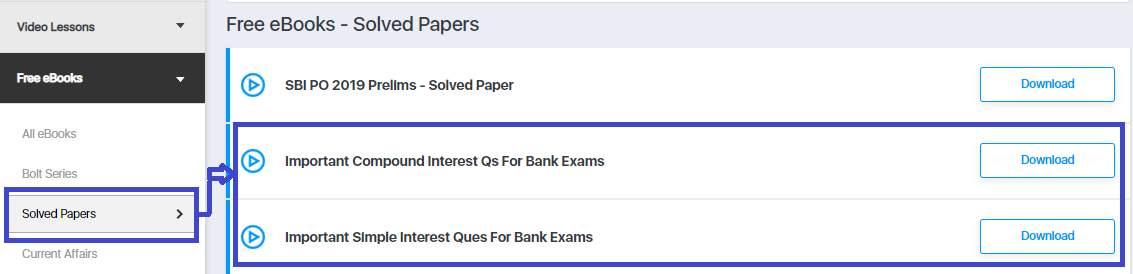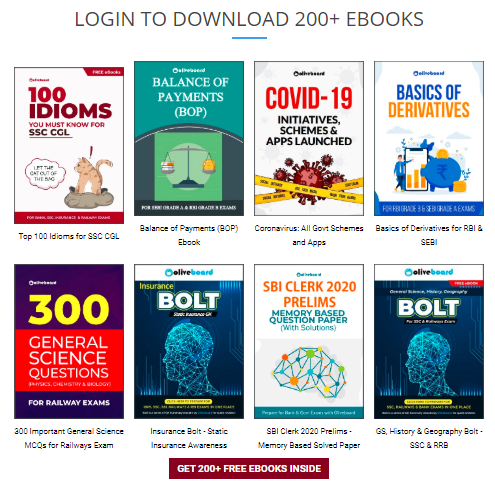# Simple Interest and Compound Interest Problems for Bank Exams

Simple and compound interest questions are not the hardest topics in quantitative aptitude, but one needs to be good with logic to answer them correctly. The same formula can be required to be inserted in various forms or ways. From the past years’ exam trends, we have uncovered that there are different types of questions that can come from the simple and compound interest chapter for your banking examinations.

Therefore, in this blog, we have brought you a free ebook on Simple and Compound Interest Questions important for upcoming banking exams. Download the free ebook now and also attempt a free mock test and practice more SI & CI questions.

Want To Take A Free Mock Test For IBPS PO? Register Here

## Compound Interest Questions | Free eBook## Simple Interest Questions | Free eBookStep 1: Click on the above-given download link. You will be taken to Oliveboard’s FREE Ebooks Page.Step 2: Register/Login on to the Free E-Books Page of Oliveboard (It is 100% free, You just enter your valid email id and a password to be able to download the Important Simple Interest & Compound Interest Practice Questions.

Step 3: After Logging in, you will see the list of Free Ebooks.

Step 4: Click on the Solved Papers section under All ebooks sections.Step 5: You will be able to download the Important Simple Interest & Compound Interest Practice Questions.

## Sneak Peek Into Free eBook

Q1) A portion of \$6600 is invested at a 5% annual return, while the remainder is invested at a 3% annual return. If the annual income from the portion earning a 5% return is twice that of the other portion, what is the total income from the two investments after one year?

A. 200
B. 270
C. 250
D. 280

Q2) An automobile financier claims to be lending money at the simple interest, but he includes the interest every six months for calculating the principal. If he is charging an interest of 10%, the effective rate of interest becomes:

A. 10%
B. 10.25%
C. 10.10%
D. 10.80%

Test Your Preparation Here By Attempting A Free Mock Test.

Q3) Ramesh invested an amount of Rs. 13,900 divided into two different schemes A and B at the simple interest rate of 14% p.a. and 11% p.a. respectively. If the total amount of simple interest earned in 2 years be Rs. 3508, what was the amount invested in Scheme B?

A. 6400
B. 6500
C. 7200
D. 7500

Q4) If the simple interest on a certain sum of money for 4 years at 5% per annum is the same as the simple interest on Rs. 560 for 10 years at a rate of 4% per annum then the sum of money is:

A. 1180
B. 1120
C. 1200
D. 1250

Check Your All India Standing. Test Your Preparation Here By Attempting A Free Mock Test.

Q5) Rahul invested a certain amount in three different schemes A, B, and C with the rate of interest 10% p.a., 12% p.a. and 15% p.a. respectively. If the total interest accrued in one year was Rs. 3200 and the amount invested in Scheme C was 150% of the amount invested in Scheme A and 240% of the amount invested in Scheme B, what was the amount invested in Scheme B?

A. Rs 5000
B. Rs 6500
C. Rs 8000
D. Rs 10000